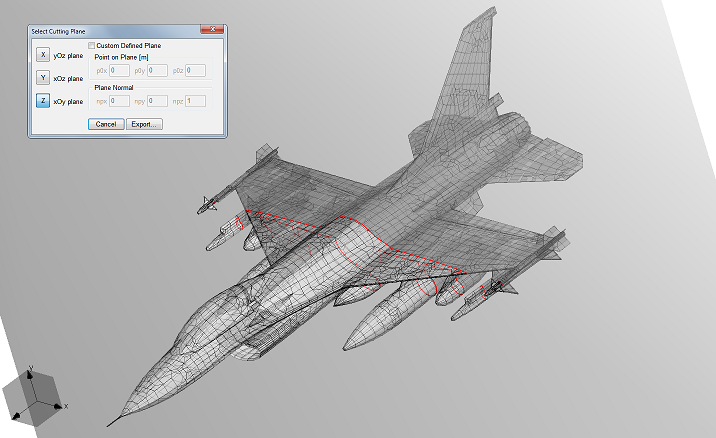WIPL-D 2D Solver is intended for numerical EM analysis of electrically very long cylindrical structures.

3D EM analysis of electrically long structures with constant cross section can be replaced with 2D analyses of its cross section. This analysis can be performed by WIPL-D 2D Solver.Example of a 2D cross-section (cut) automatically obtained from a complex 3D geometry

Interface

WIPL-D 2D Solver is integrated with WIPL-D Pro, and WIPL-D Pro CAD. Arbitrary cross-section from a 3D model can be easily extracted for 2D electromagnetic analysis. An example of a complex 3D structure with a tool for extraction of cross-section cuts is shown above. It shows how the cut is extracted from the model of a fighter airplane, while the corresponding cut imported in 2D is shown in the next figure.The tool is also integrated with WIPL-D Optimizer and WIPL-D Time Domain Solver.

The Engine

Numerical engine is based on surface integral equation formulation: electric field integral equations (EFIE) for metallic structures, and PMCHWT formulation for dielectric and magnetic materials. The engine can handle arbitrary combinations of piecewise linear materials along with any combination of infinitely or finitely thin layers with distributed loadings. Every parameter, including material and distributed loading specifications, can be frequency dependent.

WIPL-D 2D Solver uses both, in-core (matrix in RAM), and out-of-core (matrix at hard disks), matrix factorization algorithms. For in-core solution with 32 GB of RAM, analysis of cross-sections up to 6,000 wavelengths can be done in several hours.

2D kernel uses WIPL-D GPU out-of-core solver for matrix inversion. This results in significant acceleration of simulation of electrically large problems. On a machine equipped with 4 state-of-the art GPUs (nVIDIA GTX 1080 Ti, for example) analysis of cross-sections of 70,000 wavelengths can be performed in 1 hour. Analysis of cross-section of 200,000 wavelengths can be performed in 1 day.

Application Areas

2D Solver is intended for scattering and antenna array analyses. In that sense, there are two types of excitation supported in 2D solver:

• Uniform plane wave,
• Cylindrical wave, produced by far-field sources.

Both, TE and TM, polarizations are supported.